James Gregory (1638 - 1675)

From `A Short Account of the History of Mathematics' (4th edition, 1908) by W. W. Rouse Ball.

James Gregory, born at Drumoak near Aberdeen in 1638, and died at Edinburgh in October 1675, was successively professor at St. Andrews and Edinburgh. In 1660 he published his Optica Promota, in which the reflecting telescope known by his name is described. In 1667 he issued his Vera Circuli et Hyperbolae Quadratura, in which he shewed how the areas of the circle and hyperbola could be obtained in the form of infinite convergent series, and here (I believe for the first time) we find a distinction drawn between convergent and divergent series. This work contains a remarkable geometrical proposition to the effect that the ratio of the area of any arbitrary sector of a circle to that of the inscribed or circumscribed regular polygons is not expressible by a finite number of terms. Hence he inferred that the quadrature of the circle was impossible; this was accepted by Montucla, but it is not conclusive, for it is conceivable that some particular sector might be squared, and this particular sector might be the whole circle. This book contains also the earliest enunciation of the expansions in series of sin x, cos x,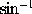x or arc sin x, and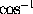x or arc cos x. It was reprinted in 1668 with an appendix, Geometriae Pars, in which Gregory explained how the volumes of solids of revolution could be determined. In 1671, or perhaps earlier, he established the theorem that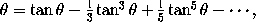the result being true only if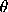lie between -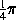and. This is the theorem on which many of the subsequent calculations of approximations to the numerical value of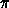have been based.

This page is included in a collection of mathematical biographies taken from A Short Account of the History of Mathematics by W. W. Rouse Ball (4th Edition, 1908).

Transcribed by

D.R. Wilkins
(dwilkins@maths.tcd.ie)
School of Mathematics
Trinity College, Dublin